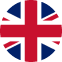Risk Committee — Nibble Financeen

# Risk Committee

The Risk Committee meets at the end of each month to determine the current interest rates for the Balanced and Special strategies.

### The Risk Committee includes the heads of the following divisions of the IT Smart Finance group of companies:

• Risk Director
• Chief Operating Officer
• Head of Underwriting Department
• Head of Debt Collection Department
• Risk Analyst

## The main tasks of the Committee:

Reviewing and analysing the results of work on NPL 30-60-90-120 for the reporting month

Reconciliation in the period of actual indicators with planned ones

Scoring model analysis

Making a forecast for the next period and calibrating the scoring model

Formation and approval of the current annual rate of return for the Balanced and Special strategies

Results of the Risk Committee meeting

Month/Year
Classic Strategy
Balanced Strategy
Special Strategy
December 2020
Approve the fixed annual interest rate of 6.5%
Approve the annual interest rate of 14,23%
Approve the annual interest rate of 17,2%
Increase the fixed annual interest rate from 6.5% to 9.7%
Increase the annual interest rate from 14.23% to 14.5%
Leave the annual interest rate unchanged at 17.2%
February 2021
Leave the annual interest rate unchanged at 9.7%
Leave the annual interest rate unchanged at 14.5%
Leave the annual interest rate unchanged at 17.2%
March 2021
Leave the annual interest rate unchanged at 9.7%
Leave the annual interest rate unchanged at 14.5%
Leave the annual interest rate unchanged at 17.2%
April 2021
Leave the annual interest rate unchanged at 9.7%
Leave the annual interest rate unchanged at 14.5%
Leave the annual interest rate unchanged at 17.2%
May 2021
Leave the annual interest rate unchanged at 9.7%
Leave the annual interest rate unchanged at 14.5%
Leave the annual interest rate unchanged at 17.2%
June 2021
Leave the annual interest rate unchanged at 9.7%
Leave the annual interest rate unchanged at 14.5%
Leave the annual interest rate unchanged at 17.2%
July 2021
Leave the annual interest rate unchanged at 9.7%
Leave the annual interest rate unchanged at 14.5%
Leave the annual interest rate unchanged at 17.2%
August 2021
Leave the annual interest rate unchanged at 9.7%
Leave the annual interest rate unchanged at 14.5%
Leave the annual interest rate unchanged at 17.2%
September 2021
Leave the annual interest rate unchanged at 9.7%
Leave the annual interest rate unchanged at 14.5%
Leave the annual interest rate unchanged at 17.2%